# Population Dynamics of Soybeans

2842 words (11 pages) Essay in Biology

25/05/18 Biology Reference this

Disclaimer: This work has been submitted by a student. This is not an example of the work produced by our Essay Writing Service. You can view samples of our professional work here.

Any opinions, findings, conclusions or recommendations expressed in this material are those of the authors and do not necessarily reflect the views of UK Essays.

Study of Population Dynamics of Soybean Semilooper Gesonia gemma by using Rule Induction Model in Maharashtra

• Cruz Antony J1 and Pratheepa M2*

### ABSTRACT

Gesonia gemma Swinhoe (1885), the grey semilooper has emerged as a serious threat to the soybean crop. This defoliator causes heavy damage to the crop in the form of loss in grain weight and grain number. Gesonia gemma population from various districts of Maharashtra has been taken to study the population dynamics related to abiotic features. Sequential covering algorithm (CN2 rule induction) has been proposed for rule induction model to generate a list of classification rules with target feature (G. gemma population) and the independent abiotic features. The classification rules have exhibited more accuracy and can be used to study the collected evidence for prediction.

KEYWORDS: CN2 rule induction, Gesonia gemma, population dynamics, semilooper, soybean

## INTRODUCTION

Soybean [Glycine max (L.) Merrill] belonging to the family Fabaceae commonly known as legume family as it is widely grown for its edible bean which has a good amount of calcium, protein and vitamins. In early seventies the crop was considered to be the safer crop against the insect pests. However, the scenario has changed as researchers from many parts of India have confirmed that seed yield and seed quality are being adversely affected by major insect pests viz. girdle beetle, tobacco caterpillar, grey semilooper, Helicoverpa armigera, jassids and white fly Netam et al. (2013).

Gesonia gemma Swinhoe (1885), the grey semilooper has emerged as a serious threat to the soybean crop. This insect pest causes defoliation to the crop, which inflicts significant reduction in pod number, pod weight, grain number and grain weight, resulting loss of grain 3.94q/ha, Yadav et al. (2014). Studying the population dynamics and forecasting the pest (G. gemma) population using the collected evidence, will bring out a successful module in Integrated Pest Management (IPM) on soybean.

Rule induction model has been proposed to understand population dynamics, since the rules (IF-THEN) are said to be more expressive and human readable in nature. One of the sequential covering algorithm, CN2 rule induction is used in building the model. CN2 induction algorithm, Clark and Niblett (1989) is a collective work based on AQ algorithm and ID3 algorithm. It is developed to classify rules accurately even in the presence of noise and the rules are made to be efficient and simple. CN2 classification rules have shown 93.73% accuracy in heart disease prediction, a comparative study done by Ramaraj and Thanamani. (2013). The proposed rule induction model will extract IF-THEN rules by classifying of pest population as high, medium and low based on Economic Threshold Level (ETL) on soybean.

## MATERIALS AND METHODS

### Dataset

The dataset comprises of field pest scouting data from various districts of Maharashtra viz., Akola, Amravati, Nagpur, Wardha and Yavatmal, along with its respective abiotic factors which includes maximum temperature (MaxT), minimum temperature (MinT), relative humidity (RH), moisture adequacy index (MAI), soil moisture index (SMI), total rainfall (RF) and number of rainfall days in a week (RFD) from 2009 to 2013. The sample data is given in the below table

 S.No PI (Larval count/ m row) SW CS MaxT (°C) MinT (°C) RH (%) MAI SMI RF (mm) RFD 1 2.22 27 1 28.75 25.33 77.50 100 72 7.70 1 2 2.34 28 1 28.31 25.00 81.67 100 82 85.40 4 3 3.61 29 1 27.17 23.67 89.58 100 100 89.90 4 4 2.68 30 2 27.34 23.67 89.33 100 100 25.30 1 5 1.31 31 2 28.13 23.90 85.25 91 83 20.80 1 6 0.73 32 2 29.18 23.65 80.50 83 72 4.50 1 7 0.53 33 3 30.19 24.25 81.25 68 60 32.70 3 8 2.33 34 3 31.12 25.50 88.75 100 99 202.60 7 9 2.28 35 4 28.85 24.33 90.50 100 100 131.80 7 10 1.92 36 4 27.69 23.94 87.75 100 100 131.40 7 11 10.62 37 4 29.36 24.41 82.25 98 84 24.60 3

Table 1. Sample tuples from the dataset

PI – pest incidence; SW – standard week; CS – crop stage; MaxT – maximum temperature; MinT – minimum temperature; RH – relative humidity; MAI – moisture adequacy index; SMI – soil moisture index; RF – rainfall in mm; RFD – number of rainfall days in a week;

### Data preprocessing

The pest population count has been classified as low, medium and high based upon the Economic Threshold Level (ETL), and it has been defined using standard week and crop stage (pre-flowering and post-flowering).

Table 2. Classification of PI according to the proposed ETL

 Crop stage Standard week Low Medium High Pre-flowering 27 to 33 PI < 3.0 PI >3.0 to 5.9 PI > 6.0 Post-flowering 34 to 41 PI < 2.5 PI > 2.5 to 4.9 PI > 5.0

The data discretization method was used to convert the continuous features (MaxT, MinT, RH, MAI, SMI, RF) into categorical counterparts. Max-diff discretization method has been chosen upon binning method, since it is highly non redundant in nature while binning the features. This method calculates the maximum of all the differences between each tuple of their respective feature and categorizes accordingly for binning the features. The pseudo code for the max-diff discretization method is given below

procedure maxdiff(data : no. of tuples, diff : no. of differences between tuples, pos : no. of position of max. difference between tuples)

//Ascending order

n = length(data)

repeat

newn = 0

for i=0 to n-1 inclusive do

if data[i-1] > data[i] then

swap(data[i-1], data[i])

newn = i

end if

end for

n = newn

until n = 0

//Max. Difference

for j = 1 to n inclusive do

diff[j-1] = data[j]-data[j-1]

end for

//Position of Max. Difference

d = length(diff)

max = diff

for k=0 to bin-1 inclusive do

for l=0 to d-1 inclusive do

if diff[l] > max then

max = diff[l]

pos[k] = l

end if

end for

end for

end procedure

Using this Max-Diff method the continuous variables are categorized into discrete variables of 5 bins (A1, A2, A3, A4, A5) each except the feature (no. of rainfall days).

### CN2 rule induction model

Rules (IF-THEN rules) are the most expressive and human readable representations for learned hypotheses, Anderson and Moore (1998). Rule induction models basically allows the interrelationships of features to influence the output from the classification, Chu and Lin (2005). Hence they are said to be sensitive than that of other classifiers which considers features as independent to the target feature. The Rule induction uses the following CN2 classifier procedure for extracting rules from the dataset:

population = Orange.data.Table(“population”)

// Construct the learning algorithm and use it to induce a classifier

cn2_classifier = Orange.classification.rules.CN2Learner(population)

// Printing all rule-base classifiers

for r in cn2_classifier.rules:

print Orange.classification.rules.rule_to_string(r)

Using the CN2 classifier five sets of IF-THEN rules have been generated for the five different districts of Maharashtra.

## RESULTS

The IF-THEN rules derived from CN2 classifier for five districts of Maharashtra from 2009 to 2013 are shown in below figures (Figure 1 to 5).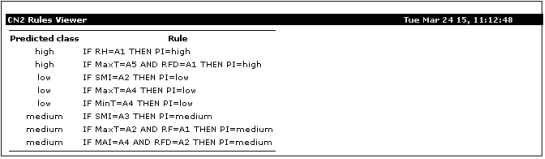MaxT – A2 = 27.60 0C to 28.70 0C; A4 = 35.30 0C to 35.50 0C; A5 = 35.86 0C to 36.14 0C, MinT – A4 = 25.00 0C, RH – A1 = 73.3%, RF – A1 = 0.0 mm to 144.0 mm, RFD – A1 = 0; A2 = 1, MAI – A4 = 80 % to 84%, SMI – A2 = 15% to 18%; A3 = 40% to 44%. Figure 1. CN2 classifier – IF-THEN rules for Akola district from 2009 to 2013.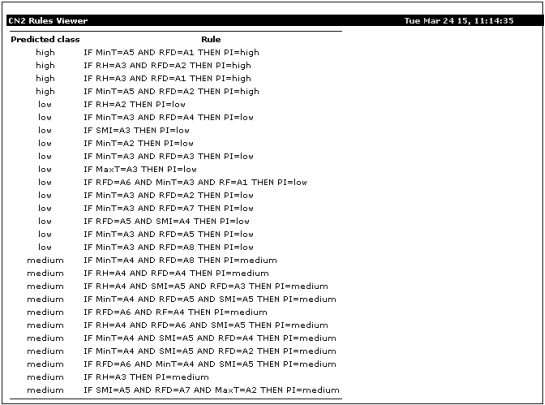MaxT – A2 = 26.29 0C to 32.46 0C; A3 = 33.00 0C, MinT – A2 = 20.43 0C to 20.46 0C; A3 = 20.70 0C to 22.50 0C; A4 = 22.75 0C to 24.00 0C; A5 = 24.50 0C, RH – A2 = 73.8% to 75.0%; A3 = 78.0%; A4 = 81.7% to 86.4%, RF – A1 = 0 mm to 244.0 mm; A4 = 315.8 mm to 328.2 mm, RFD – A1 = 0; A2 = 1; A3 = 2; A4 = 3; A5 = 4; A6 = 5; A7 = 6; SMI – A3 = 54% to 55%; A4 = 60% to 76%; A5 = 82% to 100%. Figure 2. CN2 classifier – IF-THEN rules for Amravati district from 2009 to 2013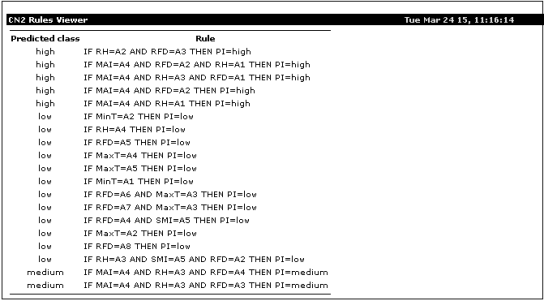MaxT – A2 = 28.10 0C; A3 = 29.50 0C to 33.70 0C; A4 = 35.29 0C; A5 = 35.83 0C, MinT – A1 = 19.71 0C; A2 = 22.29 0C to 22.40 0C, RH – A1 = 76.7% to 77.1%; A2 = 78.0% to 80.8%; A3 = 82.6% to 84.0%; A4 = 85.0%, RFD – A1 = 0; A2 = 1; A3 = 2; A4 = 3; A5 = 4; A6 = 5; A7 = 6; A8 = 7; MAI – A4 = 94% to 97%; SMI – A5 = 89% to 100%. Figure 3. CN2 classifier – IF-THEN rules for Nagpur district from 2009 to 2013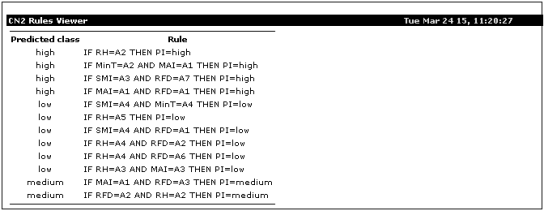MinT – A2 = 18.80 0C to 20.00 0C; A4 = 21.71 0C to 23.94 0C, RH – A2 = 75.0%; A3 = 77.5% to 80.0%; A4 = 82.0% to 88.0%; A5 = 90.0% to 96.6%, RFD – A1 = 0; A2 = 1; A3 = 2; A7 = 6, MAI – A1 = 75%; A3 = 85% to 87%, SMI – A3 = 50% to 71%; A4 = 77% to 94%. Figure 4. CN2 classifier – IF-THEN rules for Wardha district from 2009 to 2013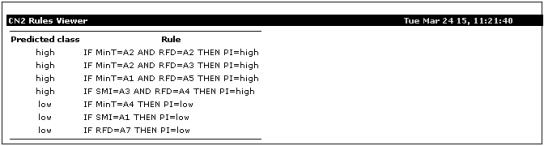MinT – A1 = 18.00 0C; A2 = 18.80 0C to 20.00 0C; A4 = 21.71 0C to 23.94 0C, RFD – A2 = 1; A3 = 2; A4 = 3; A5 = 4; A7 = 6, SMI – A1 = 16%; A3 = 50% to 71%. Figure 5. CN2 classifier – IF-THEN rules for Yavatmal district from 2009 to 2013

The IF-THEN rules derived from CN2 classifier reveals that in Akola district, when MaxT is between 35.86 0C to 36.14 0C and with no rainfall days (RFD = 0) the pest incidence (PI) was high. Similarly, when RH is 73.3 the PI was high.

In Amravati district, when MinT is 24.50 0C and with RFD is 0 or RFD is 1, the PI was high. Similarly, when RH is 78.0% and with RFD is 0 or RFD is 1, the PI was high.

In Nagpur district, when RH is between 78.0% to 80.8% and with RFD is 2, the PI was high. When MAI is between 94% to 97%, RH is between 76.7% to 77.1% and with RFD is 0 or RFD is 1, the PI was high. And when MAI is between 94% to 97% and RH is between 76.7% to 77.1% or RFD is 1, the PI was high.

In Wardha district, when RH is 75.0%, the PI was high. When MinT is between 18.80 0C to 20.00 0C and with MAI is 75%, the PI was high. When SMI is between 50% to 71% and with RFD is 6, the PI was high. And when MAI is 75% and with RFD is 0, the PI was high.

In Yavatmal district, when MinT is between 18.80 0C to 20.00 0C and with RFD is 1 or RFD is 2, the PI is high. When MinT is 18.00 0C and with RFD is 4, the PI was high. And when SMI is between 50% to 71% and RFD is 3, the PI was high.

## CONCLUSION

Studying the population dynamics of G.gemma in Maharashtra has been successfully done by using the CN2 classification rules. The derived rules have exhibited clearly that higher temperature and lower rainfall are the favorites of pest population. But when lower the temperature and more the rainfall days then the population seems to be very less, considering all five important districts of Maharashtra. Thus the rule induction model makes it easy to study the population dynamics of G. gemma occurring on soybean, which will help in the development of better prediction module in IPM in time for sustainable agriculture.

## REFERENCES

Brigham Anderson and Andrew Moore. (1998). ADtrees for Fast Counting and for Fast Learning of Association Rules. Knowledge Discovery from Databases ’98.

Clark, P. and Niblett, T. (1989). The CN2 induction algorithm. Machine Learning 3(4):261-283.

Netam, H.K, Gupta, R and Soni, S. (2013). Seasonal incidence of insect pests and their biocontrol agents on soybean. IOSR-JAVS, (2): 07-11.

Ramaraj, M and Thanamani, A.S. (2013). A comparative study of CN2 rule and SVM algorithm and prediction of heart disease datasets using clustering algorithms. Network and Complex Systems, 3(10): 1-7.

Sharma, M.C. (2001). Integrated Pest Management in developing countries: Special Reference to India, IPM Mitr, 37-44.

Wesley Chu and Tsau Young Lin. (2005). Foundations and Advances in Data Mining. Springer Science & Business Media.

Yadav, S.S., M.K. Nayak, A.K. Srivastava, M.P. Gupta and D.S. Tomar. (2014). Population dynamics of insect defoliator of soybean and correlation with weather parameters. Ann. Pl. Protec. Sci. (22): 208-209.

If you need assistance with writing your essay, our professional essay writing service is here to help!

## Related Services

View all

### DMCA / Removal Request

If you are the original writer of this essay and no longer wish to have the essay published on the UK Essays website then please:Prices from
###### £124

Undergraduate 2:2 • 1000 words • 7 day delivery

Delivered on-time or your money back

##### Rated 4.6 out of 5 by(178 Reviews)